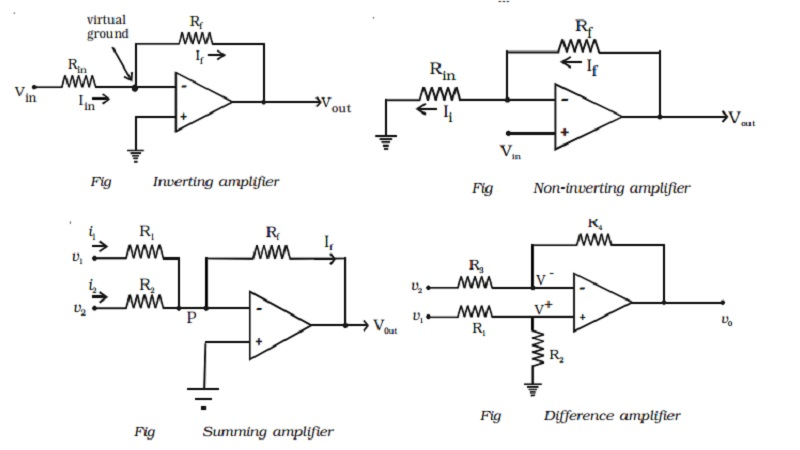Home | | Physics | | Physics | Basic OP-AMP circuits: Inverting, Non-inverting, Summing, Difference amplifier

# Basic OP-AMP circuits: Inverting, Non-inverting, Summing, Difference amplifierBasic OP-AMP circuits : This section concentrates on the principles involved with basic OP-AMP circuit viz, (i) inverting and (ii) non-inverting amplifiers. (i) Inverting amplifier (ii) Non-inverting amplifier (iii) Summing amplifier (iv)Difference amplifier

Basic OP-AMP circuits

This section concentrates on the principles involved with basic OP-AMP circuit viz, (i) inverting and (ii) non-inverting amplifiers.

(i) Inverting amplifier

The basic OP-AMP inverting amplifier is shown in Fig. The input voltage Vin is applied to the inverting input through the input resistor Rin. The non inverting input is grounded. The feedback resistor Rf is connected between the output and the inverting input.

Since the input impedance of an op-amp is considered very high, no current can flow into or out of the input terminals. Therefore Iin must flow through Rf and is indicated by If (the feedback current). Since Rin and Rf are in series, then Iin = If. The voltage between inverting and non-inverting inputs

is essentially equal to zero volt. Therefore, the inverting input terminal is also at 0 volt. For this reason the inverting input is said to be at virtual ground. The output voltage (Vout) is taken across Rf.

It can be proved that

If=Vout/Rf

Since Iin =If , then

Vin/Rin = -Vout/Rf

Rearranging the equation, we obtain

-Vout/Vin = Rf/Rin

The voltage gain of an inverting amplifier can be expressed as

Av = -Rf/Rin

The amplifier gain is the ratio of Rf  to Rin

Finally, the output voltage can be found by

Vout = -Rf/Rin x Vin

The output voltage is out of phase with the input voltage.

(ii) Non-inverting amplifier

The basic OP-AMP non-inverting amplifier is shown in Fig. The input signal Vin is applied to the non-inverting input terminal. The resistor Rin is connected from the inverting input to ground. The feedback resistor Rf is connected between the output and the inverting input.

Resistors Rf and Rin form a resistive ratio network to produce the feedback voltage (VA) needed at the inverting input. Feedback voltage (VA) is developed across Rin. Since the potential at the inverting input tends to be the same as the non-inverting input (as pointed out with the description of virtual ground), Vin = VA.

Since VA = Vin, the gain of the amplifer can be expressed as

Av  = Vou tV A

However, VA is determined by the resistance ratio of Rin and Rf ; thus,

VA=[Rin/(Rf/Rin)] Vout

Av=1+(Rf/Rin)

Finally, the output voltage can be found by, Vout = (1+Rf/Rin)Vin

It is seen that the input and output voltages are in phase(iii) Summing amplifier

The summing amplifier provides an output voltage equal to the algebraic sum of the input voltages.

Fig shows an inverting amplifier, used to sum two input voltages. The input voltages v1 and v2 are applied through the resistors R1 and R2 to the summing junction (P) and Rf is the feedback resistor. At the point P,

i1 + i2 =if

Since the voltage at the point P is ideally 0

(V1/R1) + (V2/R2) =(Vout/Rf)

Hence the output voltage,

Vout=-[(RfV1/R1) +(RfV2/R2) ]

If R1 = R2 = Rf  = R, then vout = - (v1 + v2)

Hence the output voltage is equal to the sum of the input voltages and the circuit acts as a summing amplifier. The negative sign indicates that OP-AMP is used in the inverting mode.(iv)Difference amplifier

The difference amplifier is shown in Fig. The output voltage can be obtained by using superposition principle. To find the output voltage v01 due to v1 alone, assume that v2 is shorted to ground. Then

V+ = R2V1 / R1+R2

Therefore, with both inputs present, the output is

V0 = V01 + V02

= (R3+R4 / R3)    (R2/R1+R2)  V1    -    (R4/R3)V2

If R1 = R2 = R3 = R4 = R

then vo = v1 - v2

If all the external resistors are equal, the voltage difference amplifier functions as a voltage subtractor.

Study Material, Lecturing Notes, Assignment, Reference, Wiki description explanation, brief detail

Related Topics